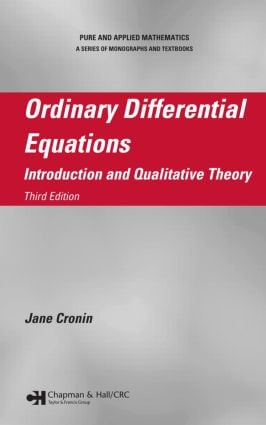Ordinary Differential Equations

Introduction and Qualitative Theory, Third Edition, 3rd Edition

CRC Press

Purchasing Options:\$ = USD
Paperback: 9780367387969
pub: 2019-09-05
SAVE ~\$14.99
\$74.95
\$59.96
x
Hardback: 9780824723378
pub: 2007-12-14
SAVE ~\$26.00
\$130.00
\$104.00
x
eBook (VitalSource) : 9780429136191
pub: 2007-12-14
from \$37.48

FREE Standard Shipping!

Description

Designed for a rigorous first course in ordinary differential equations, Ordinary Differential Equations: Introduction and Qualitative Theory, Third Edition includes basic material such as the existence and properties of solutions, linear equations, autonomous equations, and stability as well as more advanced topics in periodic solutions of nonlinear equations. Requiring only a background in advanced calculus and linear algebra, the text is appropriate for advanced undergraduate and graduate students in mathematics, engineering, physics, chemistry, or biology.

This third edition of a highly acclaimed textbook provides a detailed account of the Bendixson theory of solutions of two-dimensional nonlinear autonomous equations, which is a classical subject that has become more prominent in recent biological applications. By using the Poincaré method, it gives a unified treatment of the periodic solutions of perturbed equations. This includes the existence and stability of periodic solutions of perturbed nonautonomous and autonomous equations (bifurcation theory). The text shows how topological degree can be applied to extend the results. It also explains that using the averaging method to seek such periodic solutions is a special case of the use of the Poincaré method.

Reviews

… a classic treatment of many of the topics an instructor would want in such a course, with particular emphasis on those aspects of the qualitative theory that are important for applications to mathematical biology. … A nice feature of this edition is an extended and unified treatment of the perturbation problem for periodic solutions. … a solid graduate-level introduction to ordinary differential equations, especially for applications. …

MAA Reviews, August 2010

Prefaces

Introduction

Existence Theorems

What This Chapter Is About

Existence Theorem by Successive Approximations

Differentiability Theorem

Existence Theorem for Equation with a Parameter

Existence Theorem Proved by Using a Contraction Mapping

Existence Theorem without Uniqueness

Extension Theorems

Examples

Linear Systems

Existence Theorems for Linear Systems

Homogeneous Linear Equations: General Theory

Homogeneous Linear Equations with Constant Coefficients

Homogeneous Linear Equations with Periodic Coefficients: Floquet Theory

Inhomogeneous Linear Equations

Periodic Solutions of Linear Systems with Periodic Coefficients

Sturm–Liouville Theory

Autonomous Systems

Introduction

General Properties of Solutions of Autonomous Systems

Orbits near an Equilibrium Point: The Two-Dimensional Case

Stability of an Equilibrium Point

Orbits near an Equilibrium Point of a Nonlinear System

The Poincaré–Bendixson Theorem

Application of the Poincaré–Bendixson Theorem

Stability

Introduction

Definition of Stability

Examples

Stability of Solutions of Linear Systems

Stability of Solutions of Nonlinear Systems

Some Stability Theory for Autonomous Nonlinear Systems

Some Further Remarks Concerning Stability

The Lyapunov Second Method

Definition of Lyapunov Function

Theorems of the Lyapunov Second Method

Applications of the Second Method

Periodic Solutions

Periodic Solutions for Autonomous Systems

Stability of the Periodic Solutions

Sell’s Theorem

Periodic Solutions for Nonautonomous Systems

Perturbation Theory: The Poincaré Method

Introduction

The Case in which the Unperturbed Equation Is Nonautonomous and Has an Isolated Periodic Solution

The Case in which the Unperturbed Equation Has a Family of Periodic Solutions: The Malkin–Roseau Theory

The Case in which the Unperturbed Equation Is Autonomous

Perturbation Theory: Autonomous Systems and Bifurcation Problems

Introduction

Using the Averaging Method: An Introduction

Introduction

Periodic Solutions

Almost Periodic Solutions

Appendix

Ascoli’s Theorem

Principle of Contraction Mappings

The Weierstrass Preparation Theorem

Topological Degree

References

Index

Exercises appear at the end of each chapter.

Cronin, Jane